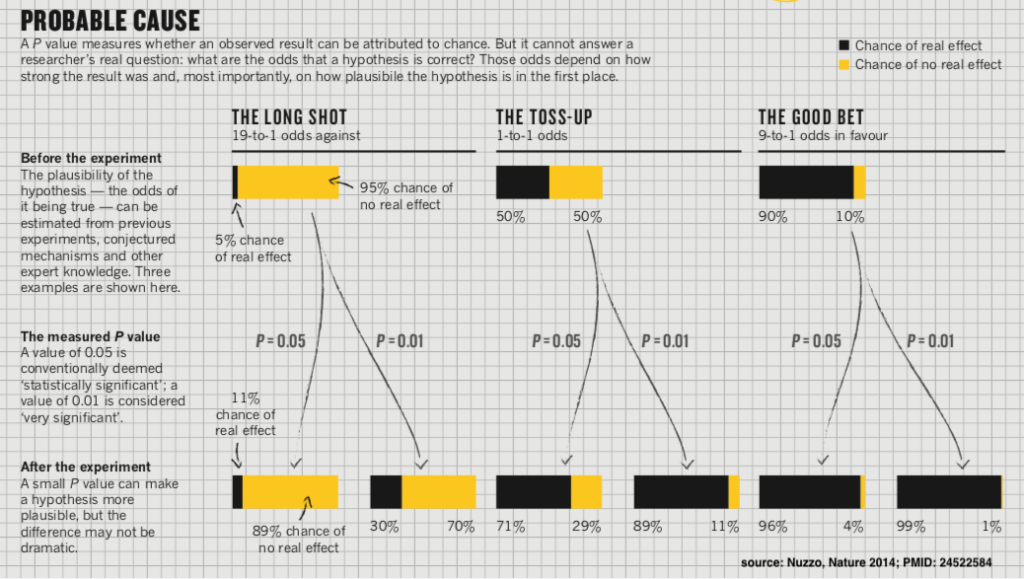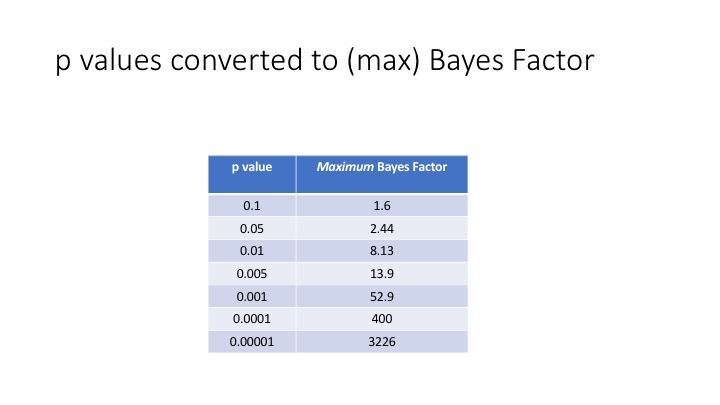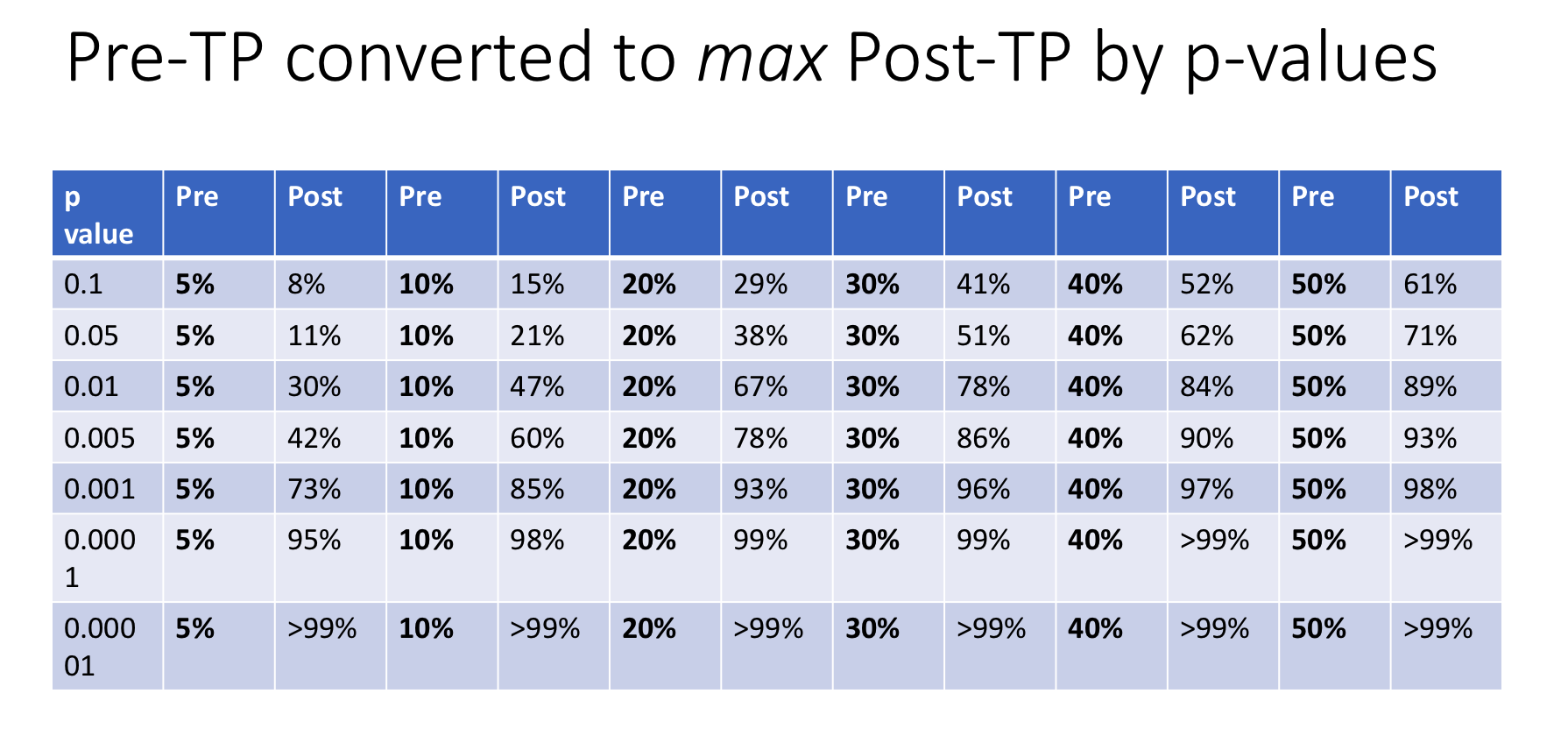# Converting p Values Into the Probability That a Finding is Real

This is a supplementary post to the EBM 2.0 project – you may wish to start there, if you haven’t already.

There it was revealed that the common belief that p values (i.e 1 minus the p value) tell us the likelihood that a study finding is true was in fact a serious and dangerous misunderstanding.  Instead it was revealed that p values were effectively “likelihood ratios” or “Bayes Factors” that convert pre-test probabilities (Pre-TP) that a studied finding is true into post-test probabilities (Post-TP) where the study in question is effectively the “test” and the p value attempts to represent the accuracy of this “test”. So the key to determining the meaning of a p value for a given finding, is first attempting to estimate what you believe the pre-test probability was before the study, based on biological plausibility and prior research.

In the landmark article by Nuzzo (Nature 2014), this was beautifully represented in the following infographic.Benjamin & Berger (American Statistician 2019) have provided recent guidance in converting p values into Bayes Factors (essentially likelihood ratios) that allow clinicians to calculate Post-TP from Pre-TP, as demonstrated in the above infographic.

Of note they are referred to as Bayes Factor Bounds which means they are “maximum” possible Bayes Factors for a given p value, so should be considered the very “best case scenario” for Post-TP. In addition, if there is any bias in the study, this affects the validity of the p value calculation so any Post-TP calculated using these flawed p values are likely to be substantial over-estimates.

So use this process as an optimistic guide only and heavily discount your figures or reject the p values entirely if you suspect or determine any bias in the studies. Finally, never rely on the p values from a single study. An iron clad rule of EBM is the need for independent reproducibility of the data before you believe it, even if the Post-TP calculated from a single study’s p-value is appealing.Bayes Factors can be multiplied by pre-test odds to provide post-test odds. However probabilities are somewhat more intuitive and familiar to clinicians and converting probabilities (pre-test) to odds, applying Bayes Factor and then converting back to probabilities (post-test) adds unnecessary intellectual hassle when online calculators already exist that more simply calculate Post-TP from Pre-TP using likelihood ratios (like this one). Using these Bayes Factors and entering them into the calculators as likelihood ratios, reveals the following estimates of the absolute maximum Post-TP given a Pre-TP and p-value.### Terminology

The use of the terms “pre-test probability” and “post-test probability” are very useful for clinicians to understand and conceptualise this approach as they may already be comfortable with these terms from their use in the understanding of the accuracy/benefits of medical testing. However in future posts, we may use these terms interchangeably with equivalent terms used more widely in statistics, “prior probability” and “posterior probability”.

We are still finding our way in the world of EBM 2.0. We appreciate any feedback, comments or contributions below and collaboration via Contact Us

Warm Regards,

Dr Anand Senthi